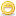1. ##kbps/Kbps/KBps....?

There seems to be a lot of confusion about the use of the abbreviations kbps/Kbps/KBps/kBps..., not only on those forums, but also on other sites. This is an attempt to get things straight...

- b or B?

b = bit, B = byte (8 bits). No confusion about that one...

A nibble is half a byte (4 bits).

- k or K?

k = kilo, K = kilo. Confused...

The explanation is easy:

k = 10 to the power of 3 = 1.000
K = 2 to the power of 10 = 1.024

--> kb = kilobit = 1.000 bits = 125 bytes
--> Kb = kilobit = 1.024 bits = 128 bytes
--> kB = kilobyte = 1.000 bytes = 8.000 bits
--> KB = kilobyte = 1.024 bytes = 8.192 bytes

--> A V.90 is a 56k modem, not a 56K modem...

The same goes for mega

m = 10 to the power of 6 = 1.000.000
M = 2 to the power of 20 = 1.048.576

And giga:

g = 10 to the power of 9 = 1.000.000.000
G = 2 to the power of 30 = 1.073.743.824

I hear you coming already: 'So what? The difference isn't THAT big!'
Take a look at this: you buy a '30 gigabyte' HDD (that's what it says on the box). You install the huge mother****er, and in your BIOS it says: 28 GB.... Ha! HDD manufactureres use the g-prefix, but computers use the G-prefix (because computers use the binary system, HDD manufacturers prefer to use the decimal system...).

--> 30 gB (the manufacturer's point of view) : 30 times 1 billion= 30.000.000.000 bytes
--> 30 GB (your computer's point of view): 30 times 2 to the power of 30 = 32.212.314.720 bytes

Meaning: if you need a 30 GB HDD, you'll need to buy a 32 GB HDD. A 30 GB HDD simply isn't big enoughI know some of you won't agree: there's another theory stating that prefixes BELOW zero should not have capitals, and prefixes above zero take a capital. I don't see why that should be, because none of the below-zero prefixes are the same as the above-zero ones....2. I know what I was thinking....holy hell....what did he just say? I need to start reading more. Useful post though. Thanx. Peace.3. ##Thank's for the info.4. ##info, goodie..

Thank you for this post, very informitive and it will definately be helpful later on.
As for the other upper- and lowercase theory, that refers to normal physical measurements in the metric system (ie, how much water? How long? etc.), and the main reason for the differences are that the shortened versions of some of the labels are the same without the case differences (ie megameter(Mm and above 0) vs. millimeter(mm and below 0)).####Posting Permissions

• You may not post new threads
• You may not post replies
• You may not post attachments
• You may not edit your posts
•